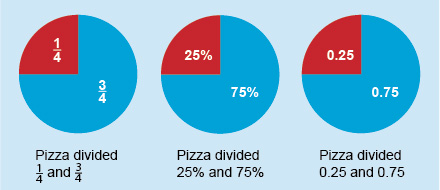Succeed with maths – Part 1

Start this free course now. Just create an account and sign in. Enrol and complete the course for a free statement of participation or digital badge if available.

Free course

# 1.1 Writing a percentage as a fraction or decimal

Fractions, decimals and percentages are all interchangeable, so you can choose to use whichever is most appropriate for your situation. From the definition of a percentage, ‘75 per cent’ means 75 out of 100. This can therefore be written as , which can be simplified to Alternatively, means , or 0.75. Therefore, .

So to write a percentage as a fraction, the numerator for the fraction is the percentage value and the denominator is always 100. Because a fraction can also be viewed as an instruction to divide by the denominator, which is 100, in order to write a percentage as a decimal you should divide it by 100.

Understanding how to represent percentages as a fraction or a decimal will help a great deal when you carry out calculations involving percentages – so getting to grips with this now is time well spent. As well as carrying out the activities this week, you can give yourself extra practice by converting percentages that you see in your everyday life.

You can show this visually by comparing three pizzas divided using percentages, fractions and finally decimals. From this it is clear that and , or 25% and 75%, or 0.25 and 0.75, always amounts to the same thing.Figure _unit6.1.1 Figure 1 Pizza maths

When writing a percentage as a decimal, you have a shortcut to the answer. Dividing a number by 100 has the same effect as moving its decimal point two places to the left.

To check that this works, enter 6.0 (the equivalent of 6 per cent) into your calculator and divide by 100.

6.0 ÷ 100 = 0.06, and the decimal point has indeed moved two places to the left.

Remember, the decimal point can be inserted at the end of your number behind the units place when dealing with a whole number.

So, .

What about writing decimals as percentages? You’ll look at how to do this in the next section.